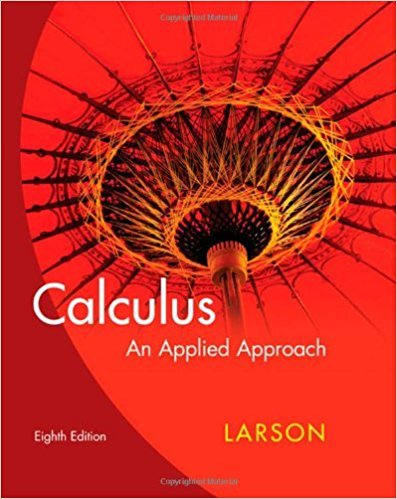×
×

# Solutions for Chapter 1: Functions, Graphs, and Limits## Full solutions for Calculus: An Applied Approach | 8th Edition

ISBN: 9780618958252Solutions for Chapter 1: Functions, Graphs, and Limits

Solutions for Chapter 1
4 5 0 240 Reviews
26
5
##### ISBN: 9780618958252

Calculus: An Applied Approach was written by and is associated to the ISBN: 9780618958252. Since 121 problems in chapter 1: Functions, Graphs, and Limits have been answered, more than 22591 students have viewed full step-by-step solutions from this chapter. This expansive textbook survival guide covers the following chapters and their solutions. Chapter 1: Functions, Graphs, and Limits includes 121 full step-by-step solutions. This textbook survival guide was created for the textbook: Calculus: An Applied Approach , edition: 8.

Key Calculus Terms and definitions covered in this textbook
• Algebraic model

An equation that relates variable quantities associated with phenomena being studied

• Amplitude

See Sinusoid.

• Common difference

See Arithmetic sequence.

• Difference of functions

(ƒ - g)(x) = ƒ(x) - g(x)

• Elimination method

A method of solving a system of linear equations

• Equivalent systems of equations

Systems of equations that have the same solution.

• General form (of a line)

Ax + By + C = 0, where A and B are not both zero.

• Graph of a function ƒ

The set of all points in the coordinate plane corresponding to the pairs (x, ƒ(x)) for x in the domain of ƒ.

• Integers

The numbers . . ., -3, -2, -1, 0,1,2,...2

• Inverse properties

a + 1-a2 = 0, a # 1a

• Local maximum

A value ƒ(c) is a local maximum of ƒ if there is an open interval I containing c such that ƒ(x) < ƒ(c) for all values of x in I

• Parametric equations

Equations of the form x = ƒ(t) and y = g(t) for all t in an interval I. The variable t is the parameter and I is the parameter interval.

• Parametrization

A set of parametric equations for a curve.

• Perihelion

The closest point to the Sun in a planet’s orbit.

• Permutation

An arrangement of elements of a set, in which order is important.

• Pythagorean

Theorem In a right triangle with sides a and b and hypotenuse c, c2 = a2 + b2

• Right-hand limit of ƒ at x a

The limit of ƒ as x approaches a from the right.

• Secant line of ƒ

A line joining two points of the graph of ƒ.

• Third quartile

See Quartile.

• Vector

An ordered pair <a, b> of real numbers in the plane, or an ordered triple <a, b, c> of real numbers in space. A vector has both magnitude and direction.

×